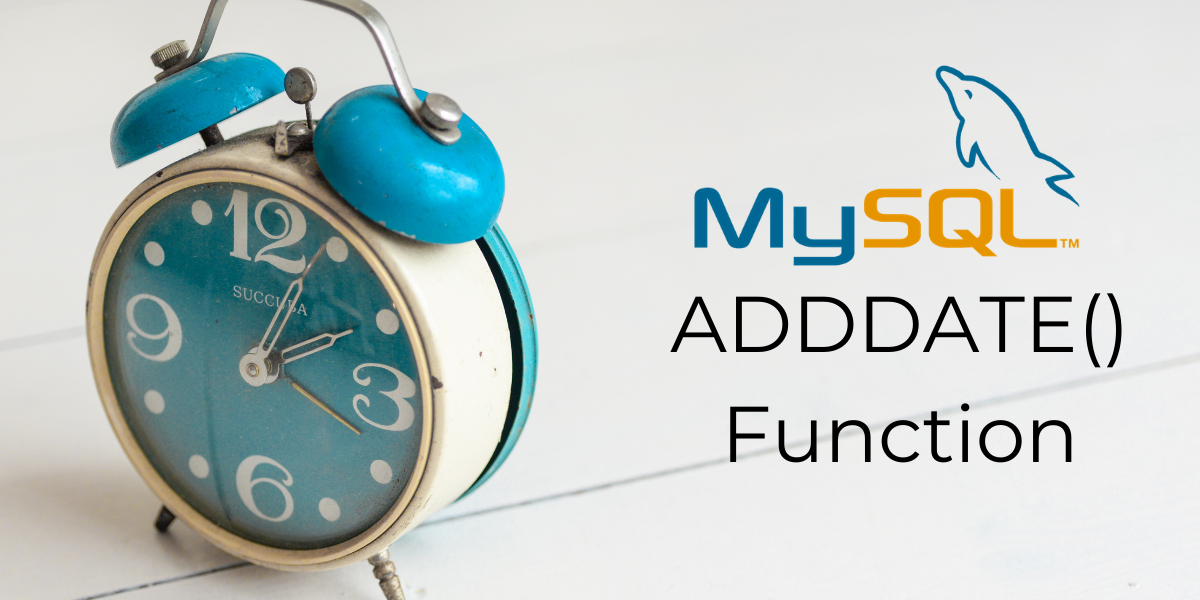In this tutorial, we will study the MySQL `ADDDATE()` function. Suppose you are an HR manager and you have been given a table which contains the details of every employee in the company.

Details include their ID, Name, joining date, department, email, salary, and office code. The company has a policy of offering an initial contract of 4 years and 6 months to every employee.

The COO of the company has asked you to add that value to the joining date and create another column in the table that specifies the contract expiration date. This is a situation where we can use the `ADDDATE()` function.

The `MySQL ADDDATE()` function is used to add a date or time interval to a date value. Let us take a look at its syntax, followed by some examples.

```ADDDATE(date, days)
```

Or,

```ADDDATE(date, INTERVAL value interval_type)
```

Where,

• ‘date’ is the date/datetime value to be modified,
• ‘days’ is the number of days to be added to ‘date’,
• ‘value’ is the value of the time interval to be added and,
• ‘interval_type’ is the type of interval to be added.

The ‘interval_type’ parameter can have the below values –

• MICROSECOND
• SECOND
• MINUTE
• HOUR
• DAY
• WEEK
• MONTH
• QUARTER
• YEAR

The below ‘interval_type’ values should be in quotes.

• SECOND_MICROSECOND where value is of the format – ‘SECONDS.MICROSECONDS’
• MINUTE_MICROSECOND where value is of the format – ‘MINUTES:SECONDS.MICROSECONDS’
• MINUTE_SECOND where value is of the format – ‘MINUTES:SECONDS’
• HOUR_MICROSECOND where value is of the format – ‘HOURS:MINUTES:SECONDS.MICROSECONDS’
• HOUR_SECOND where value is of the format – ‘HOURS:MINUTES:SECONDS’
• HOUR_MINUTE where value is of the format – ‘HOURS:MINUTES’
• DAY_MICROSECOND where value is of the format – ‘DAYS HOURS:MINUTES:SECONDS.MICROSECONDS’
• DAY_SECOND where value is of the format – ‘DAYS HOURS:MINUTES:SECONDS’
• DAY_MINUTE where value is of the format – ‘DAYS HOURS:MINUTES’
• DAY_HOUR where value is of the format – ‘DAYS HOURS’
• YEAR_MONTH where value is of the format – ‘YEARS-MONTHS’

Let us see an example of `MySQL ADDDATE()` with the first syntax from the syntax section. Consider the below queries. In the first query, we add 15 days to the given date value. In the second query, we will add 45 days to the given date value. We will use the `SELECT` statement and aliases to make our output readable.

```SELECT ADDDATE('2021-05-15', 15) AS Result;
```

And the output is –

### Adding intervals with value types

Next, let us see an example of `ADDDATE()` with the second syntax I mentioned in the syntax section. Consider the below queries.

```SELECT ADDDATE('2020-03-12', INTERVAL 1 YEAR) AS Result;
SELECT ADDDATE('2020-03-12', INTERVAL 5 DAY) AS Result;
```

In the first query, we add an interval of 1 year to the given date. In the second query, we add an interval of 5 days to the given date. The output is –

Let us see an example of `ADDDATE()` with the `CURDATE()` function. Let us write a query in which we add the current date with 3 days, 3 weeks, 3 months, 3 quarters and 3 years. Each value should be displayed separately in the result-set. The query is –

```SELECT CURDATE() AS Today,
ADDDATE(CURDATE(), INTERVAL 3 DAY) AS '+3 Days',
ADDDATE(CURDATE(), INTERVAL 3 WEEK) AS '+3 Weeks',
ADDDATE(CURDATE(), INTERVAL 3 MONTH) AS '+3 Months',
ADDDATE(CURDATE(), INTERVAL 3 QUARTER) AS '+3 Quarters',
ADDDATE(CURDATE(), INTERVAL 3 YEAR) AS '+3 Years';
```

And the output is –

### MySQL ADDDATE() With Datetime Values Using NOW()

Let us see an example with datetime values returned by the `NOW()` function. Let us write a query in which we add 50 microseconds, 50 seconds, 50 minutes, 50 hours, and 20 days 12 hours to the current date and time. Each value should be displayed separately in the result-set. The query is –

```SELECT NOW() AS 'Current Time',
ADDDATE(NOW(), INTERVAL 50 MICROSECOND) AS '+50 Microseconds',
ADDDATE(NOW(), INTERVAL 50 SECOND) AS '+50 Seconds',
ADDDATE(NOW(), INTERVAL 50 MINUTE) AS '+50 Minutes',
ADDDATE(NOW(), INTERVAL 50 HOUR) AS '+50 Hours',
ADDDATE(NOW(), INTERVAL '20 12' DAY_HOUR) AS '+20 Days and 12 Hours';
```

And the output is –

### Working with Tables

Consider the below Employee table.

Now let us get back to the problem I mentioned in the beginning. Suppose you are an HR manager and you have been given an Employee table which contains the details of every employee in the company. Now, the company has a policy of offering an initial contract of 4 years and 6 months to every employee.

The COO of the company has asked you to add that value to the joining date and create another column in the table that specifies the contract expiration date. Let us use the `ALTER` and `UPDATE` statements along with the `ADDDATE()` function. Consider the below queries.

```ALTER TABLE Employee ADD ContractEndDate date;
UPDATE Employee SET ContractEndDate=ADDDATE(Date_Joined, INTERVAL '4-6' YEAR_MONTH);
SELECT * FROM Employee;
```

We use the `ALTER` statement to add the column `ContractEndDate`. Next, using the `UPDATE` statement along with the `ADDDATE()` function to add the contract expiration dates. Note that we have used the ‘interval_type’ parameter as `YEAR_MONTH`. The 4 in the ‘value’ parameter is the number of years to be added while the 6 indicates the number of months to be added. We get the output as –

## Conclusion

The MySQL `ADDDATE()` function is very useful. Basic addition operations on date and datetime values are very important and have a plethora of use cases. I would encourage you to practice some more queries with this function.